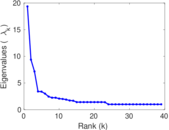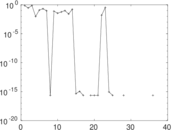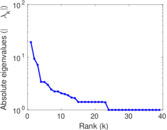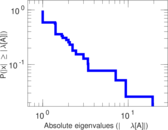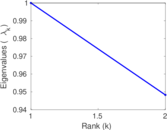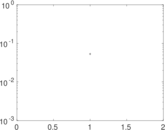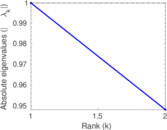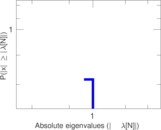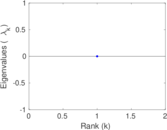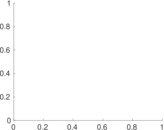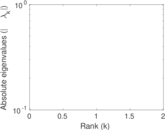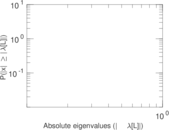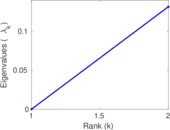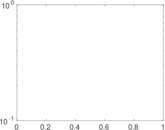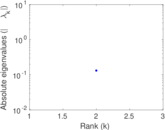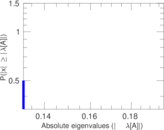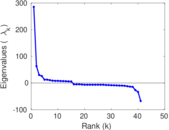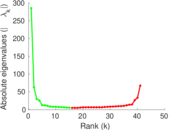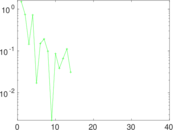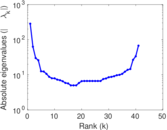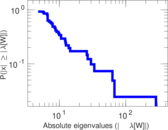# Wiktionary edits (ab)

This is the bipartite edit network of the Abkhazian Wiktionary. It contains users and pages from the Abkhazian Wiktionary, connected by edit events. Each edge represents an edit. The dataset includes the timestamp of each edit.

 Code `mab` Internal name `edit-abwiktionary` Name Wiktionary edits (ab) Data source http://dumps.wikimedia.org/ AvailabilityDataset is available for download Consistency checkDataset passed all tests Category Authorship network Dataset timestamp 2017-10-20 Node meaning User, article Edge meaning Edit Network formatBipartite, undirected Edge typeUnweighted, multiple edges Temporal dataEdges are annotated with timestamps

## Statistics

 Size n = 289 Left size n1 = 41 Right size n2 = 248 Volume m = 383 Unique edge count m̿ = 276 Wedge count s = 7,827 Claw count z = 220,366 Cross count x = 4,679,773 Square count q = 74 4-Tour count T4 = 32,796 Maximum degree dmax = 176 Maximum left degree d1max = 176 Maximum right degree d2max = 4 Average degree d = 2.650 52 Average left degree d1 = 9.341 46 Average right degree d2 = 1.544 35 Fill p = 0.027 144 0 Average edge multiplicity m̃ = 1.387 68 Size of LCC N = 170 Diameter δ = 4 50-Percentile effective diameter δ0.5 = 1.942 46 90-Percentile effective diameter δ0.9 = 3.779 73 Median distance δM = 2 Mean distance δm = 2.900 01 Gini coefficient G = 0.589 162 Balanced inequality ratio P = 0.280 679 Left balanced inequality ratio P1 = 0.154 047 Right balanced inequality ratio P2 = 0.396 867 Relative edge distribution entropy Her = 0.806 971 Power law exponent γ = 6.618 42 Tail power law exponent γt = 2.931 00 Tail power law exponent with p γ3 = 2.931 00 p-value p = 0.011 000 0 Left tail power law exponent with p γ3,1 = 1.801 00 Left p-value p1 = 0.096 000 0 Right tail power law exponent with p γ3,2 = 3.811 00 Right p-value p2 = 0.008 000 00 Degree assortativity ρ = −0.331 248 Degree assortativity p-value pρ = 1.719 34 × 10−8 Spectral norm α = 19.339 8 Spectral separation |λ1[A] / λ2[A]| = 2.061 96 Controllability C = 209 Relative controllability Cr = 0.723 183

## Plots

### Fruchterman–Reingold graph drawing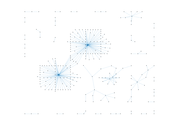### Degree distribution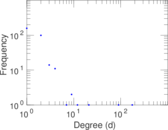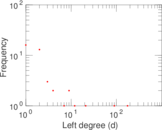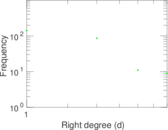### Cumulative degree distribution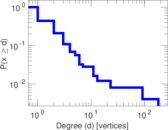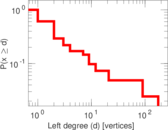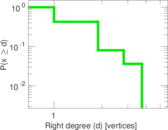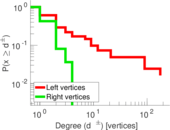### Lorenz curve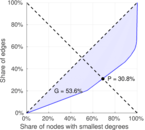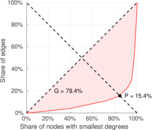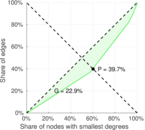### Spectral distribution of the adjacency matrix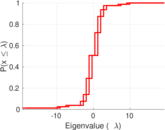### Spectral distribution of the normalized adjacency matrix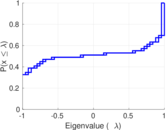### Spectral distribution of the Laplacian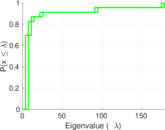### Spectral graph drawing based on the adjacency matrix### Spectral graph drawing based on the normalized adjacency matrix### Degree assortativity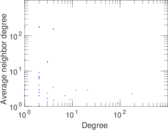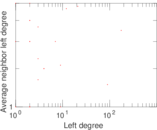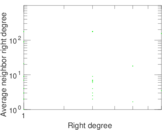### Zipf plot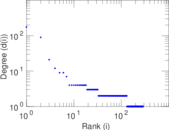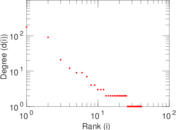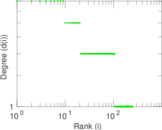### Hop distribution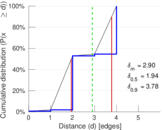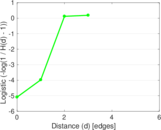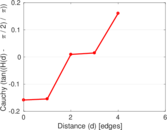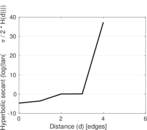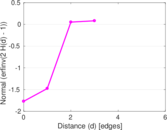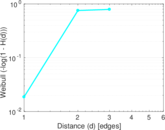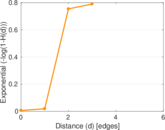### Double Laplacian graph drawing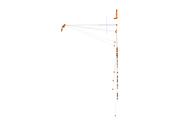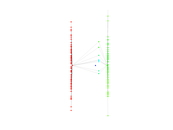### Delaunay graph drawing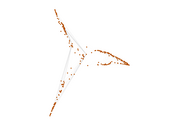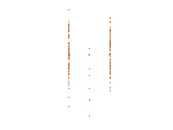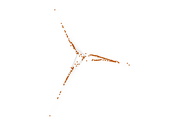### Edge weight/multiplicity distribution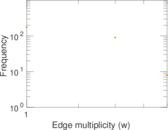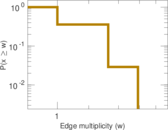### Temporal distribution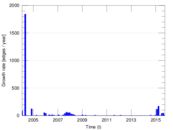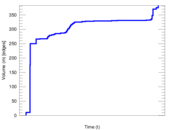### Temporal hop distribution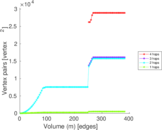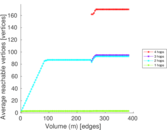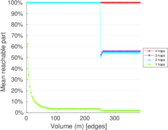### Diameter/density evolution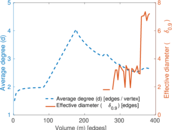### Matrix decompositions plots